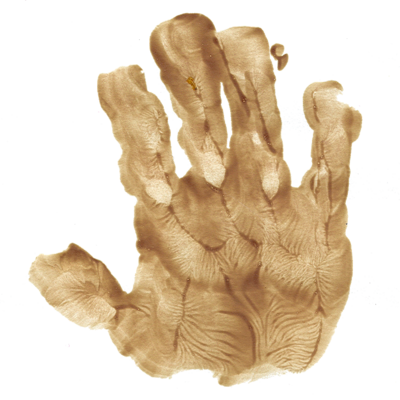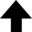I keep the subject of my inquiry constantly before me, and wait till the first dawning opens gradually, by little and little, into a full and clear light.Isaac Newton

This entry is part of a series that explores some of the properties of the planar model and the mechanism that causes the Kosterlitz-Thouless (KT) transition. The KT transition is a non-zero temperature in which a phase transition occurs in a spin lattice.Next: II. Lattice Representations

The Ising model—a case study of the Monte Carlo simulation of a canonical ensemble—is a widely-studied system of interacting variables in statistical physics. The model was proposed by Wilhelm Lenz and investigated by Ernst Ising to study the phase transition of a paramagnet to a ferromagnet. The Ising model in one dimension is visualized as a lattice of $N$ sites, where at each site is located an arrow (called a spin) that can take a value of $+1$ for "up" and $-1$ for "down". A particular configuration, or microstate, of the lattice is specified by the set of variables $\{ s_1, s_2, \dots s_N \}$ for all lattice sites. In one dimension, the total energy $E$ of the Ising model is given by $-J \sum_{i, j}^N s_i s_j - H \sum_{i = 1}^N s_i$, where the exchange constant or coupling strength $J$ is a measure of the strength of the interaction between spins $s_i$ and $s_j$, and $H$ is proportional to a uniform external magnetic field. (Note that $J \gt 0$ favors coincident arrows, or those pointed in the same direction.) The first sum is over all the nearest neighbors, while the second sum represents the total interaction energy of the magnetic moments associated with the spins $s_i$ with an external magnetic field. The thermodynamic properties of the one dimensional Ising model do not exhibit a phase transition. However, in two and three dimensions the Ising model does exhibit a phase transition, as will be shown in this series of exercises.

The planar model (also called the $x$-$y$ model) is an extension of the Ising model to two dimensions and consists of spins of unit magnitude that can point in any direction in the $x$-$y$ plane. The analytic description of this model, shown below, is expressed as a Hamiltonian that easily summarizes how spin $i$ interacts with spin $j$ through a coupling strength $J$, where $\theta_i$ and $\theta_j$ are the angles that the $i$th and $j$th spins make with their respective axes.

Part I. in this series utilizes the Monte Carlo method to simulate the planar model on a square lattice using periodic boundary conditions. The use of periodic boundary conditions is a common way of minimizing surface effects and therefore simulating the properties of a bulk system. This method is a novel way to duplicate a basic unit in the planar model an infinite number of times to include all of two dimensional space.

## I. Mean Magnetization

This program utilizes the Monte Carlo method to simulate the planar model on a square lattice using periodic boundary conditions.
$\displaystyle{ E = -J \sum_{i, j} \cos( \theta_i - \theta_j ) }$
 Number of Monte Carlo steps: Number of equilibrium steps: Value of the coupling strength $J$:
0%
Current data point: